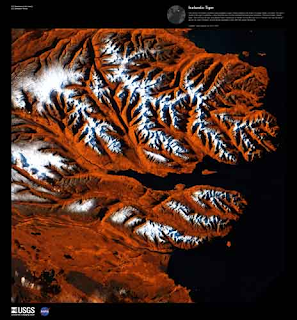## Saturday, April 28, 2012By one measurement, the Earth has 217,490 miles of coastline.

For comparison, the average distance between the Earth and the Moon is roughly the same (238,854 miles). 217,490 miles is a large, but comforting measurement.

Humans have an affinity for naming and measuring. From measuring grows understanding. Yet measurement also gives us a deceptive sense of mastery.

There's more.
The edge of the sea is a strange and beautiful place.
~ Rachel CarsonMeasuring coastline is not as simple as it appears. The aggregated length depends on the size of the ruler.The smaller the measurement, the more coastline.
How can that be?
Measured length increases without limit as the measurement scale decreases towards zero.
Mathematician Benoît Mandelbrot discussed self-similar curves, laid groundwork for fractal dimension, and examined the Coastline Paradox in a 1967 paper published in Science.
The Coastline Paradox is that the measured length of a stretch of coastline depends on the scale of measurement. Empirical evidence suggests that the smaller the increment of measurement, the longer the measured length becomes. If one were to measure a stretch of coastline with a yardstick, one would get a shorter result than if the same stretch were measured with a 30cm (one-foot) ruler. This is because one would be laying the ruler along a more curvilinear route than that followed by the yardstick.

~ Wikipedia, How Long Is the Coast of Britain? Statistical Self-Similarity and Fractional Dimension.
Resources
1. Benoît Mandelbrot, 1967, How Long Is the Coast of Britain? Statistical Self-Similarity and Fractional Dimension. Science, New Series, Vol. 156, No. 3775. (May 5, 1967), pp. 636-638. doi:10.1126/science.156.3775.636, JSTOR.
2. Coastline Paradox, Wolfram Math World.